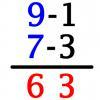#### You may also like### Route to Root

A sequence of numbers x1, x2, x3, ... starts with x1 = 2, and, if you know any term xn, you can find the next term xn+1 using the formula: xn+1 = (xn + 3/xn)/2 . Calculate the first six terms of this sequence. What do you notice? Calculate a few more terms and find the squares of the terms. Can you prove that the special property you notice about this sequence will apply to all the later terms of the sequence? Write down a formula to give an approximation to the cube root of a number and test it for the cube root of 3 and the cube root of 8. How many terms of the sequence do you have to take before you get the cube root of 8 correct to as many decimal places as your calculator will give? What happens when you try this method for fourth roots or fifth roots etc.?### Triangle Incircle Iteration

Keep constructing triangles in the incircle of the previous triangle. What happens?### Vedic Sutra - All from 9 and Last from 10

Vedic Sutra is one of many ancient Indian sutras which involves a cross subtraction method. Can you give a good explanation of WHY it works?

# Sorted

### Why do this problem?

This problem uses the context of sorting playing cards as a hook to engage students' curiosity about efficiency of different sorting algorithms.

The task could be used to introduce the idea of algorithmic complexity, by looking at how long each method takes and considering worst-case scenarios. It could also be used as a programming exercise.

By presenting many different algorithms that perform the same function, we hope students will reflect on the benefits of having a variety of tools in their mathematical toolkit when solving problems.

### Possible approach

Each student will need one suit from a pack of cards.
"Shuffle your cards, and then put them in order from Ace to King. Watch how your partner puts their cards in order. Do you both do it the same way? How efficient is your method?"

Either show students each video and invite them to make sense of the algorithm and have a go themselves,
or hand out this worksheet for them to make sense of the algorithms on paper.

"For each of the algorithms, perform it a few times to get a feel for it. Then choose two algorithms and compare them. Which is the quickest? Why? Can you put your cards into a worst-case scenario for each of the algorithms, to make it take as long as possible?"

Give students time to explore these, together with the questions from the problem which are on the worksheet:
• On average, which algorithm did you find to be quickest?
• What is the 'worst-case scenario' for each algorithm?
• How long would it take in the worst case?
Then bring the class together to discuss their findings.

### Possible extension

Invite students to use pseudocode, or a programming language if they know one, to express the algorithms.

### Possible support

Introduce the algorithms one at a time. This website shows visualisations of the different sorting algorithms which might help students to make sense of the different methods.